# Pandas – Plot multiple time series DataFrame into a single plot

• Difficulty Level : Medium
• Last Updated : 17 Oct, 2021

In this article, we are going to see how to plot multiple time series Dataframe into single plot.

If there are multiple time series in a single DataFrame, you can still use the plot() method to plot a line chart of all the time series. To Plot multiple time series into a single plot first of all we have to ensure that indexes of all the DataFrames are aligned. So let’s take two examples first in which indexes are aligned and one in which we have to align indexes of all the DataFrames before plotting.

Attention geek! Strengthen your foundations with the Python Programming Foundation Course and learn the basics.

To begin with, your interview preparations Enhance your Data Structures concepts with the Python DS Course. And to begin with your Machine Learning Journey, join the Machine Learning - Basic Level Course

## Python3

 `# importing Libraries`` ` `# import pandas as pd``import` `pandas as pd`` ` `# importing matplotlib module``import` `matplotlib.pyplot as plt``plt.style.use(``'default'``)`` ` `# %matplotlib inline: only draw static``# images in the notebook``%``matplotlib inline`

### Step 2: Importing Data

We will be plotting open prices of three stocks Tesla, Ford, and general motors, You can download the data from here or yfinance library.

Tesla file:

## Python3

 `# code``# importing Data``tesla ``=` `pd.read_csv(``'Tesla_Stock.csv'``,``                    ``index_col``=``'Date'``, ``                    ``parse_dates``=``True``)``tesla.head(``10``)`

Output: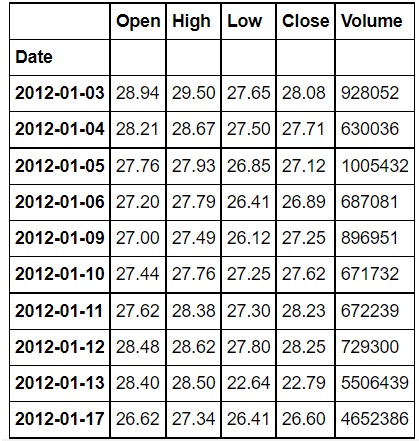Ford_stock:

## Python3

 `# code``# importing data``ford ``=` `pd.read_csv(``'Ford_Stock.csv'``,``                   ``index_col``=``'Date'``, ``                   ``parse_dates``=``True``)``ford.head(``10``)`

Output: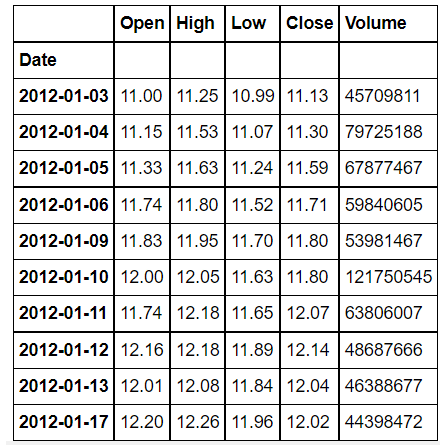GM_Stock:

## Python3

 `# code``# importing data``gm ``=` `pd.read_csv(``'GM_Stock.csv'``,``                 ``index_col``=``'Date'``,``                 ``parse_dates``=``True``)``# printing 10 entries of the data``gm.head(``10``)`

Output: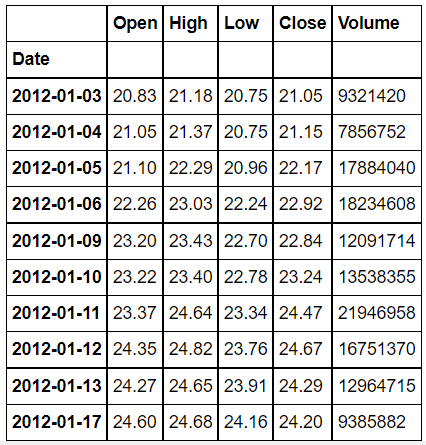## Python3

 `# code``# Visualizing The Open Price of all the stocks`` ` `# to set the plot size``plt.figure(figsize``=``(``16``, ``8``), dpi``=``150``)`` ` `# using plot method to plot open prices.``# in plot method we set the label and color of the curve.``tesla[``'Open'``].plot(label``=``'Tesla'``, color``=``'orange'``)``gm[``'Open'``].plot(label``=``'GM'``)``ford[``'Open'``].plot(label``=``'Ford'``)`` ` `# adding title to the plot``plt.title(``'Open Price Plot'``)`` ` `# adding Label to the x-axis``plt.xlabel(``'Years'``)`` ` `# adding legend to the curve``plt.legend()`

Output: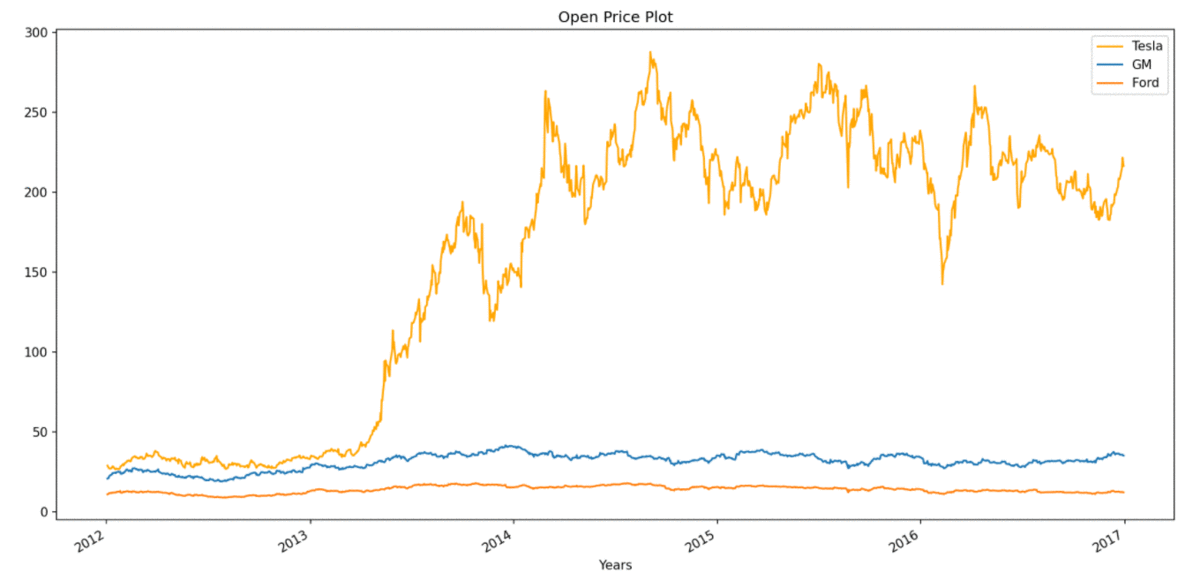## Plotting DataFrames with different DateTime Index:

In the second example, we will take stock price data of Apple (AAPL) and Microsoft (MSFT) off different periods. Our first task here will be to reindex any one of the dataFrame to align with the other dataFrame and then we can plot them in a single plot.

## Python3

 `# importing Libraries`` ` `# import pandas as pd``import` `pandas as pd`` ` `# importing matplotlib module``import` `matplotlib.pyplot as plt``plt.style.use(``'default'``)`` ` `# %matplotlib inline: only draw static images in the notebook``%``matplotlib inline`

## Python3

 `# code``aapl ``=` `pd.read_csv(``'aapl.csv'``,``                   ``index_col``=``'Date'``,``                   ``parse_dates``=``True``)``# printing 10 entries of the data``aapl.head(``10``)`

Output: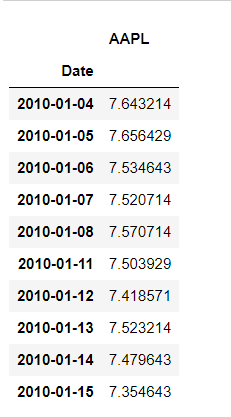msft file:

## Python3

 `# importing Data``msft ``=` `pd.read_csv(``'msft.csv'``, ``                   ``index_col``=``'Date'``,``                   ``parse_dates``=``True``)``# printing 10 entries of the data``msft.head(``10``)`

Output: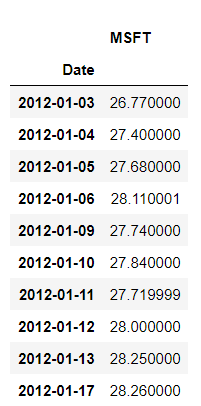As you can clearly see, DateTime index of both DataFrames is not the same, so firstly we have to align them. When we will make DateTime index of msft the same as that of all, then we will have some missing values for the period 2010-01-04 to 2012-01-02 , before plotting It is very important to remove missing values.

## Python3

 `# Aligning index``aapl[``"MSFT"``] ``=` `msft.MSFT`` ` `# removing Missing Values``aapl.dropna(inplace``=``True``)`` ` `aapl.head(``10``)`

Output: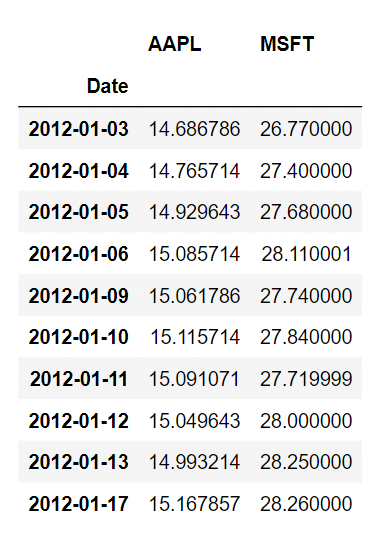We have merged the two DataFrames, into a single DataFrame, now we can simply plot it,

## Python3

 `# Visualizing The Price of the stocks``# to set the plot size``plt.figure(figsize``=``(``16``, ``8``), dpi``=``150``)`` ` `# using .plot method to plot stock prices.``# we have passed colors as a list``aapl.plot(label``=``'aapl'``, color``=``[``'orange'``, ``'green'``])`` ` `# adding title``plt.title(``'Price Plot'``)`` ` `# adding label to x-axis``plt.xlabel(``'Years'``)`` ` `# adding legend.``plt.legend()`

Output: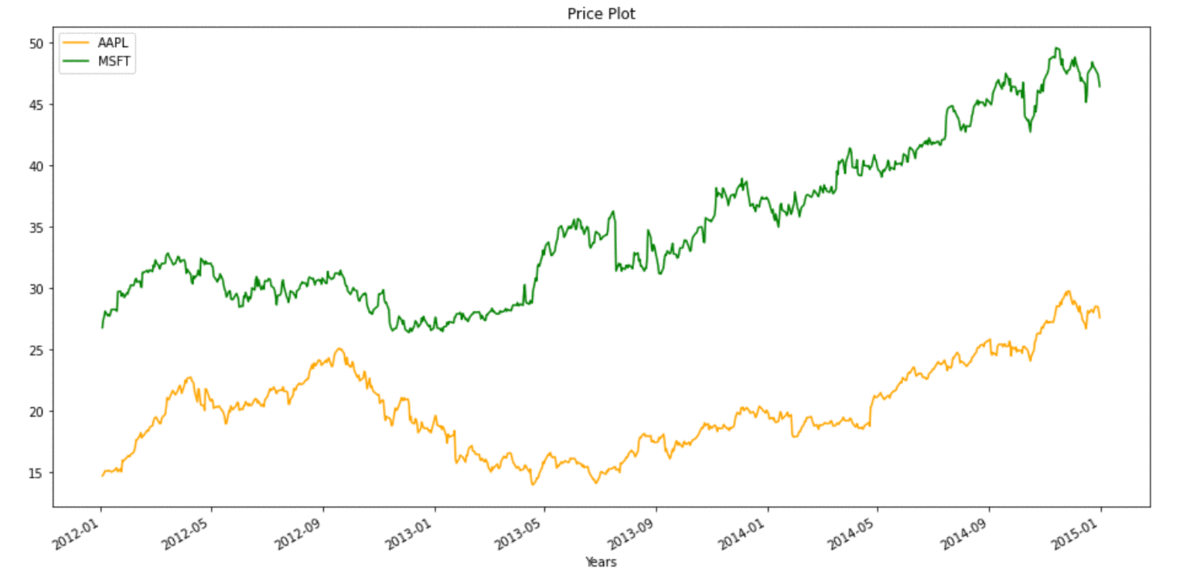In some cases we can’t afford to lose data, so we can also plot without removing missing values, plot for the same will look like: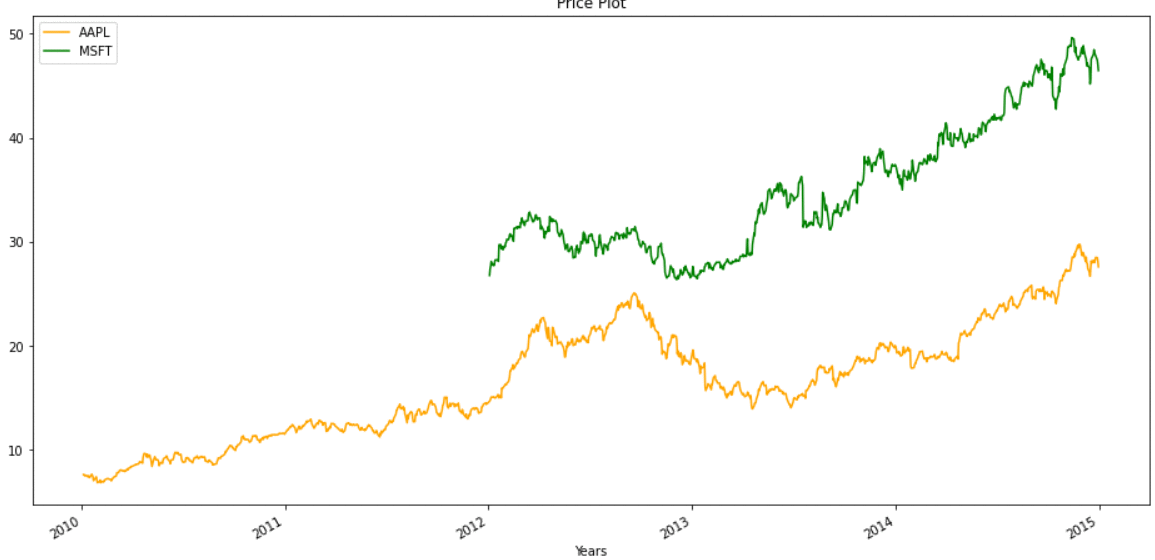My Personal Notes arrow_drop_up